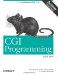# 33.CGI Programming with Perl

## 4.3. Decoding Form Input

In order to access the information contained within the form, we must decode the data that is sent to us. The algorithm for decoding form data is:

1. Read the query string from \$ENV{QUERY_STRING}.

2. If the \$ENV{REQUEST_METHOD} is POST, determine the size of the request using \$ENV{CONTENT_LENGTH} and read that amount of data from the standard input. Append this data to the data read from the query string, if present (this should be joined with "&").

3. Split the result on the " &" character, which separates name-value pairs (the format is name=value&name=value...).

4. Split each name-value pair on the "s" character to get the name and value.

5. Decode the URL-encoded characters in the name and value.

6. Associate each name with its value(s); remember that each option name may have multiple values.

A form sends its parameters as the body of a POST request, or as the query string of a GET request. However, it is possible to create a form that uses the POST method and direct it to a URL containing a query string. Thus, it is possible to get a query string with a POST request.

Here is a first attempt at our subroutine:

`sub parse_form_data {     my %form_data;     my \$name_value;     my @name_value_pairs = split /&/, \$ENV{QUERY_STRING};          if ( \$ENV{REQUEST_METHOD} eq 'POST' ) {         my \$query = "";         read( STDIN, \$query, \$ENV{CONTENT_LENGTH} ) == \$ENV{CONTENT_LENGTH}           or return undef;         push @name_value_pairs, split /&/, \$query;     }          foreach \$name_value ( @name_value_pairs ) {         my( \$name, \$value ) = split /=/, \$name_value;                  \$name =~ tr/+/ /;         \$name =~ s/%([\da-f][\da-f])/chr( hex(\$1) )/egi;                  \$value = "" unless defined \$value;         \$value =~ tr/+/ /;         \$value =~ s/%([\da-f][\da-f])/chr( hex(\$1) )/egi;                  \$form_data{\$name} = \$value;     }     return %form_data; }`

You can use parse_form_data like this:

`my %query = parse_form_data(  ) or error( "Invalid request" ); my \$activity = \$query{activity};`

We split the query string into name-value pairs and then store each pair in @name_value_pairs. Since the client puts ampersands between key-value pairs, the split command specifies an ampersand as the delimiter. If the request method is POST, then we also read the content of the request from STDIN. If the number of bytes that we read does not match the number that we expect, we return undef. This could happen if the user presses their browser's Stop button while sending a request.

We then loop over each of the name-value pairs and spit them into \$name and \$value. It is possible that a parameter can be passed without an equal sign or a value. This happens for <ISINDEX> forms, which are virtually never used anymore, or for manually constructed URLs. By setting the \$value to an empty string when it isn't defined, we avoid warnings from Perl.

We replace each + with a space character. We then decode URL-encoded characters by replacing strings that start with % and that are followed by two hexadecimal characters using the expression that we discussed in Chapter 2, "The Hypertext Transport Protocol ". We then add the name and value pair to our hash, which we return when we are done.

You may have noticed that there is a problem with our subroutine; it occurs in the hash assignment near the end of the subroutine:

`\$form_data{\$name} = \$value;`

If the form has elements that share the same name, or if there is a scrolling box that supports multiple values, then it is possible for us to receive multiple values for the same name. For example, if you choose "One" and "Two" in a select list with the variable name "numbers," the query string would look like:

`numbers=One&numbers=Two`

Our example earlier would save only the last value in the hash. There are a couple different ways we could solve this, but neither is ideal. First, we could convert the value of the hash into an array reference for multiple values by replacing the hash assignment with the following lines:

`if ( exists \$form_data{\$name} ) {     if ( ref \$form_data{\$name} ) {         push @{ \$form_data{\$name} }, \$value;     }     else {         \$form_data{\$name} = [ \$form_data{\$name}, \$value ];     } else {     \$form_data{\$name} = \$value; }`

This code is somewhat complex, but because it is hidden in our subroutine, this isn't really an issue. The real problem with this approach is that CGI scripts using this subroutine need to know which elements can have multiple values and must test each one or run the risk of mistakenly believing the user entered something like "ARRAY(0x19abcde)", which is Perl's scalar representation of an array reference. Code to access the values of the "numbers" element would look like this:

`my %query = parse_form_data(  ) or error( "Invalid request" ); my @numbers = ref( \$query{numbers} ) ? @{ \$query{numbers} } : \$query{numbers};`

This syntax is awkward. Another approach is to store the multiple values as a single text string that is delimited by a certain character, such as a tab or "\0". This is easier to code in the subroutine:

`if ( exists \$form_data{\$name} ) {     \$form_data{\$name} .= "\t\$value"; else {     \$form_data{\$name} = \$value; }`

It is also easier to read in the CGI script:

`my %query = parse_form_data(  ) or error( "Invalid request" ); my @numbers = split "\t", \$query{numbers};`

However, there is still a potential for corrupted data if the CGI script is not expecting multiple values.

Fortunately, there is a better solution. Instead of writing an input subroutine ourselves, we can use CGI.pm, which provides an effective solution to this problem along with many other useful features. The next chapter discusses CGI.pm.

 4.2. Form Tags 5. CGI.pmCGI Programming with Perl
ISBN: 1565924193
EAN: 2147483647
Year: 1999
Pages: 120

Similar book on Amazon